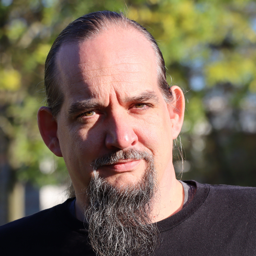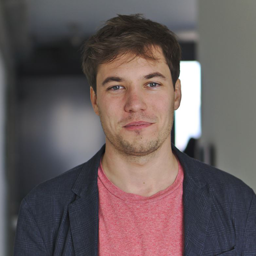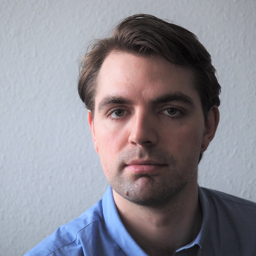# New Trends in Computational Science in Engineering and Industrial Mathematics

Europe/Berlin
Toepffer-Villa (Magdeburg)

#### Toepffer-Villa (Magdeburg)

Participants
•Alexander Zuyev
•Ali Forootani
•Anahita Iravanizad
•André Schneider
•Ashwin Nayak
•Athanasios Antoulas
•Boris Khoromskij
•Carolin Penke
•Diana Noatsch-Liebke
•Dimitrios Karachalios
•Feliks Nüske
•Harshit Kapadia
•Heike Faßbender
•Heiko Weichelt
•Hermann Mena
•Igor Pontes Duff
•Ion Victor Gosea
•Iryna Baraniukova
•Janine Holzmann
•Jennifer Przybilla
•Jens Saak
•Jingjing Zhang
•Jonas Schulze
•Judith Schneider
•Karim Cherifi
•Kathryn Lund
•Kirandeep Kour
•Leonidas Gkimisis
•Lihong Feng
•Manuel Baumann
•Martin Köhler
•Martin Redmann
•Martin Stoll
•Melina Freitag
•Neveen Eshtewy
•Nicoleta Sima
•Norman Lang
•Patrick Kürschner
•Paul Van Dooren
•Pawan Goyal
•Petar Mlinarić
•Peter Benner
•Quirin Aumann
•Rene Schneider
•Sabine Hein
•Sandeep Reddy Bukka
•Sara Grundel
•Sergey Chuiko
•Shaimaa Monem Abdelhafez
•Shuwen Sun
•Sridhar Chellappa
•Steffen W. R. Werner
•Süleyman Yildiz
•Teodor Nikolov
•Thomas Bendokat
•Thomas Mach
•Tim Mitchell
•Tobias Breiten
•Tobias K. S. Ritschel
•Vahid Nateghi
•Vasile Sima
•Venera Khoromskaia
•Yevgeniya Filanova
•Zoran Tomljanovic
Contact (information, letter of invitation)
• Friday, July 1
• 12:00 PM
Welcome reception
• Scientific Program Friday: History
• 1
CSC history

tba

Speaker: Peter Benner
• 2
SIAM Student Chapter of Magdeburg

The SIAM Student Chapter of Magdeburg is an association of students at the Otto-von-Guericke University and the Max Planck Institute in Magdeburg. Being part of the SIAM Chapter means having opportunities to develop networks with students from different faculties, departments, and research fields. This cooperation allows us to share ideas and research with people having similar interests, organize events together, learn about career options, and develop leadership skills. We will provide an introduction to the purpose and activities of the SIAM Chapter.

Speakers: Harshit Kapadia (Max Planck Institute for Dynamics of Complex Technical Systems), Yevgeniya Filanova (Max Planck Institute for Dynamics of Complex Technical Systems)
• 4:00 PM
Coffee break
• Scientific Program Friday: Numerical (Multi-)Linear Algebra
• 3
Self-conjugate linearizations of self conjugate transfer functions

We prove that we can always construct strongly minimal linearizations of an arbitrary rational matrix from its Laurent expansion around the point at infinity, which happens to be the case for polynomial matrices expressed in the monomial basis. If the rational matrix has a particular self-conjugate structure we show how to construct strongly minimal linearizations that preserve it. The structures that are considered are the Hermitian and skew-Hermitian rational matrices with respect to the real line, and the para-Hermitian and para-skew-Hermitian matrices with respect to the imaginary axis. We pay special attention to the construction of strongly minimal linearizations for the particular case of structured polynomial matrices. The proposed constructions lead to efficient numerical algorithms for constructing strongly minimal linearizations. The fact that they are valid for any
rational matrix is an improvement on any other previous approach for constructing other classes of structure preserving linearizations, which are not valid for any structured rational or polynomial matrix. The use of the re-
cent concept of strongly minimal linearization is the key for getting such generality.
Strongly minimal linearizations are Rosenbrock’s polynomial system matrices of the given rational matrix, but with a quadruple of linear polynomial matrices (i.e. pencils) :
L(λ) :=[ A(λ) −B(λ) C(λ) D(λ)],
where A(λ) is regular, and the pencils [ A(λ) −B(λ) ] and
[ A(λ) C(λ)] have no finite or infinite eigenvalues. Strongly minimal linearizations contain the complete information about the zeros, poles and minimal indices of the rational matrix and allow to recover very easily its eigenvectors and minimal
bases. Thus, they can be combined with algorithms for the generalized eigenvalue problem for computing the complete spectral information of the rational matrix.

Speaker: Paul Van Dooren (UC Louvain)
• 4
Prospects of tensor methods in numerical modeling of large bio-molecule systems

I consider the range-separated (RS) tensor format in numerical modeling of many particle interactions. The main focus is on efficient calculation of electrostatic potential of bio-molecules. Both free space potential and the case of polarized media will be addressed. In particular, the regularization of the Poisson-Boltzmann equation will be considered and numerical illustrations will be presented.

Speaker: Boris Khoromskij (Max-Planck Institute for Mathematics in the Sciences, Leipzig)
• 5
From sets of linear to sets of polynomial equations

First, I give a brief overview about some of my recent work regarding large-scale linear algebra, in particular systems of linear equations and matrix equations. Afterwards, I speak about what happens from a linear algebra viewpoint when we allow polynomial nonlinearities in the systems.

Speaker: Patrick Kürschner (HTWK Leipzig)
• 6
Adaptive Cross Approximation for Ill-Posed Problems

Ill-posed problems require regularization to be solved. That is, it is necessary to project the problem onto a suitable subspace damping the influence of small singular values. Adaptive cross approximation is capable to identify low-rank approximations and, thus, reduce the number of small singular values.

In the talk we will demonstrate that this in fact works and what is needed additionally to obtain an efficient working solver.

Chebfun uses adaptive cross approximation for computing the singular value expansion of operators. We will use Chebfun to generalize the ideas above from matrices to integral operators.

Speaker: Thomas Mach (Universität Potsdam)
• 7
Ä Tännschen, please - Matrix operations at the core of modern Natural Language Processing in OpenGPT-X

The field of Natural Language Processing (NLP) has been undergoing a revolution in recent years. Large-scale language models (LLMs), most notably a series of Generative Pre-trained Transformers (GPTs), exceeded all expectations in benchmark scenarios and real life applications such as text generation, translation, question-answering and summarization.
The engine of the NLP revolution is the so-called attention mechanism, which now allows to process longer sentences without “forgetting” important words. This mechanism is implemented in form of a series of matrix products and lends itself to intense parallelization. The pre-training of transformers requires great computational resources and is one example of the increasing AI workload of large High Performance Computing (HPC) facilities.
OpenGPT-X is a joint effort of 10 partners from science and industry to train and provide access to an open LLM based in Europe in order to guarantee its digital and economic sovereignty. Within this project, the pre-training of the LLM is performed at the Jülich Supercomputing Centre.

Speaker: Carolin Penke
• Networking
• Saturday, July 2
• Scientific program Saturday: Control
• 8
Stabilizing Dynamical Systems in the Scarce Data Regime

Stabilizing dynamical systems in science and engineering is challenging, especially in edge cases and limit states where typically little data are available. In this work, we propose a data-driven approach that guarantees finding stabilizing controllers from as few data samples as the dimension of the unstable dynamics, which typically is orders of magnitude lower than the state dimension of the system. The key is learning stabilizing controllers directly from data without learning models of the systems, which would require larger numbers of data points. Numerical experiments with chemical reactors and fluid dynamics behind obstacles demonstrate that the proposed approach stabilizes systems after observing fewer than five data samples even though the dimension of states is orders of magnitude higher.

Speaker: Steffen W. R. Werner (Courant Institute of Mathematical Sciences, New York University)
• 9
Finite time horizon mixed control of vibrational systems

We consider a vibrational system control problem over a finite time horizon. The performance measure of the system is taken to be $p$-mixed $H_2$ norm which generalizes the standard $H_2$ norm. We present an algorithm for efficient calculation of this norm in the case when the system is parameter dependent and the number of inputs or outputs of the system is significantly smaller than the order of the system. Our approach is based on a novel procedure which is not based on solving Lyapunov equations and which takes into account the structure of the system. We use a characterization of the $H_2$ norm given in terms of integrals which we solve using adaptive quadrature rules. This enables us to use recycling strategies as well as parallelization. The efficiency of the new algorithm allows for an analysis of the influence of various system parameters and different finite time horizons on the value of the $p$-mixed $H_2$ norm. We illustrate our approach by numerical examples concerning an $n$-mass oscillator with one damper.

Speaker: Zoran Tomljanovic (Department of Mathematics University of Osijek)
• 10
Stabilization of an underactuated satellite model with magnetorquers by oscillating controls

We consider the mathematical model of a low orbit satellite with electromagnetic actuation described in the recent paper [R.Misra,R.Wisniewski, A.Z. ”Attitude Stabilization of a Satellite having only Electromagnetic Actuation using Oscillating Controls”, Aerospace (submitted)]. This model is not fully actuated as the control torque is proportional to the vector product of the geomagnetic field and the field generated by magnetorquers. The case of periodic time varying geomagnetic field has been previously studied by several authors. We focus here on the case of constant geomagnetic field, which corresponds to an equatorial orbit and brings significant theoretical challenges for control design. A family of time periodic feedback controllers is proposed to stabilize the equilibrium of this model. We discuss the convergence rate of the closed-loop system and illustrate the resulting dynamics with numerical simulations.

Speaker: Alexander Zuyev
• 11
An artificial pancreas for people with type 1 diabetes based on nonlinear model predictive control

Type 1 diabetes (T1D) is a chronic disease caused by autoimmune desctruction of the pancreatic insulin-producing cells. People with T1D spend significant amounts of time on self-treatment with insulin infusion or injections. However, this task is non-trivial, and administering too much insulin can be dangerous. Conversely, administering too little insulin for longer periods of time can lead to long-term complications. We present an artificial pancreas (AP) for controlling the blood glucose concentration in people with T1D. The AP consists of 1) a sensor (a continuous glucose monitor), 2) actuators (e.g., an insulin pump), and 3) a nonlinear model predictive control algorithm implemented on a smartphone. We discuss the computational aspects of the algorithm (optimal control, state estimation, and maximum likelihood parameter estimation) as well as the clinical trial in which it is currently being tested. Finally, we present numerical results for 50 virtual people with T1D.

Speaker: Tobias K. S. Ritschel (Department of Applied Mathematics and Computer Science, Technical University of Denmark)
• 10:50 AM
Coffee break
• 12:20 PM
Lunch
• Scientific program Saturday: MOR
• 12
Is IRKA a Riemannian Optimization Method?

We consider the Riemannian submanifold of matrix-valued rational functions of fixed McMillan degree embedded in the Hardy $\mathcal{H}_2$ space and explore whether IRKA can be interpreted as a Riemannian optimization method over this manifold.

Speaker: Petar Mlinarić (Virginia Tech)
• 13
A Posteriori Error Estimation and Adaptivity for Model Order Reduction of Large-Scale Systems

We study a posteriori error estimation and adaptivity with the goal of automatic model order reduction of large-scale systems. We propose efficient offline adaptive techniques that are aimed at (a) bringing down the significant offline cost often associated with generating reduced-order models and (b) minimizing the user interference in obtaining efficient reduced-order models. Adaptivity is considered in two aspects: adaptive basis enrichment and adaptive training set sampling. The adaptive techniques we propose are enabled by efficient and sharp a posteriori error estimators. The error estimators not only guide the offline generation of reduced-order models, but also provide error certification for their online use. Our adaptive techniques cover linear steady, time-harmonic, dynamical systems and nonlinear dynamical systems. We demonstrate the advantages resulting from our approach on a range of numerical examples and finally chart out interesting avenues of future research.

Speaker: Sridhar Chellappa (Max Planck Institute for Dynamics of Complex Technical Systems)
• 14
Reducing the dynamics of deformable complex surfaces.

Simulating dynamics of deforming surfaces is very expensive, particularly when internal forces, acting on vertices and/or their connecting faces, require real-time update, and especially because the 2-dimensional objects embedded in 3D spaces are meshes with hundreds of thousands of vertices. The dynamical behavior of such structure is governed by Newton's law of motion for mechanical systems under internal and external forces, $\mathbf M \ddot{q}(t) = f_{int}(q(t))+ f_{ext}$.

Utilizing the variational formulation of the system, we can write positions $q(t) \in \mathbb{R}^{N \times 3}$ of vertices at different time steps, as a minimizer that compromises between both momentum and potential energies accompanying the mechanical structure. The computations then can be divided into many parallel local nonlinear solves and one linear global solve; this is well known as the projective dynamics scheme.

The nonlinear internal forces, such as bending and strain, express and control the material behavior of the surface as a geometrical object and they require re-computation at every time step. External forces typically remain constant during computations.

We explore tackling the computational complexity of the problem by projecting to a low dimensional reduced subspace, we consider different candidate methods, namely proper orthogonal decomposition, localized sparse-PCA and localized quaternion-PCA. We compare to skinning subspaces which have been introduced earlier in literature.

Speaker: Shaimaa Monem
• 2:20 PM
Coffee break
• Scientific program Saturday: Machine Learning & Digital Twins
• 15
Centralities for multilayer networks

Centrality measures identify and rank the most influential entities of complex networks. In this talk, we generalize matrix function-based centrality measures, which have been studied extensively for single-layer and temporal networks in recent years to layer-coupled multiplex networks. The layers of these networks can reflect different relationships and interactions between entities or changing interactions over time. We use the supra-adjacency matrix as network representation, which has already been used to generalize eigenvector centrality to temporal and multiplex networks. With a suitable choice of edge weights, the definition of single-layer matrix function-based centrality measures in terms of walks on networks carries over naturally to the multilayer case. In contrast to other walk-based centralities, matrix function-based centralities are parameterized measures, which have been shown to interpolate between (local) degree and (global) eigenvector centrality in the single-layer case. As the explicit evaluation of the involved matrix function expressions becomes infeasible for medium to large-scale networks, we present highly efficient approximation techniques from numerical linear algebra, which rely on Krylov subspace methods, Gauss quadrature, and stochastic trace estimation. We present extensive numerical studies on synthetic and real-world multiplex transportation, communication, and collaboration networks. The comparison with established multilayer centrality measures shows that our framework produces meaningful rankings of nodes, layers, and node-layer pairs. Furthermore, our experiments corroborate the theoretically indicated linear computational complexity of the employed numerical methods for sparse supra-adjacency matrices, which allows the efficient treatment of large-scale networks with the number of node-layer pairs of order 10^7 or higher.

Speaker: Martin Stoll (TU Chemnitz)
• 16
Machine Learning Approaches to Learn Dynamical Models

Dynamical modeling of a process is essential to study its dynamical behavior and perform engineering studies such as control and optimization. With the ease of accessibility of data, learning models directly from the data have recently drawn much attention. It is also desirable to construct simple and compact models describing complex nonlinear high-fidelity dynamics for efficient simulations and engineering studies on modest computer hardware. In this direction, we provide an overview of several machine learning approaches to learn dynamical systems. We demonstrate these approaches using several numerical examples.

Speaker: Pawan Goyal (Max Planck Institute for Dynamics of Complex Technical Systems)
• 17
The MPI-Magdeburg, Peter and Me
Speaker: Thanos Antoulas (Rice/MPI/Baylor)
• Social Event (Bus Sightseeing) leaving at Jakobstr near Alter Markt
• 6:30 PM
Dinner

Get together and discussions

• 18
After dinner speech
Speaker: Sara Grundel
• 9:00 PM
Networking